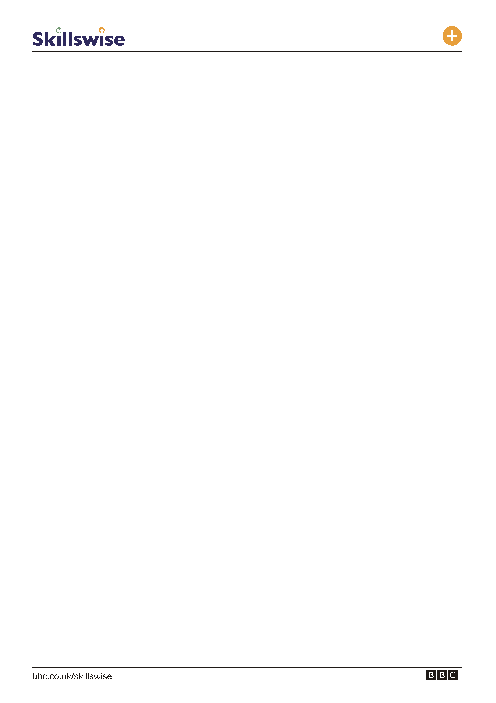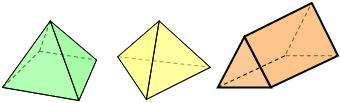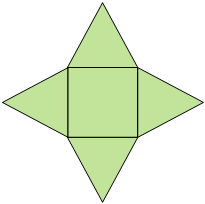# 1. Which of the following is a 3-d shape?

 Page 1/8 Date 28.06.2018 Size 236.5 Kb.## 3D shapes

L1

Level A

1. Which of the following is a 3-D shape?

1. Cylinder

2. Octagon

3. Kite

2. What is another name for 3-D shapes?

1. Polygon

2. Polyhedron

3. Point

3. A 3-D shape has four sides and a triangular face. This 3-D shape could be1. Square based pyramid

2. Triangular based pyramid

3. Triangular prism

4. Which of the following is a trianguler based pyramid?

1. Triangular polygon

2. Tetrahedron

3. Trihedron

5. A 3-D shape has been opened up to a flat 2-D shape (a net) and is shown below? What was the 3-D shape?